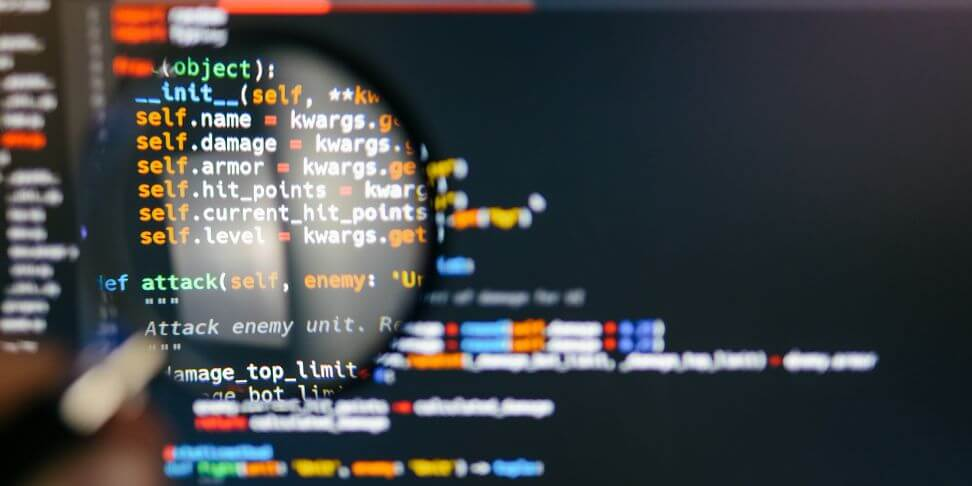How it works?FAQContact## C++ - standard library

### What it is

The C++ standard library contains a set of classes and interfaces that vastly extend the capabilities of the language. It is an invaluable help in everyday work while developing programs.

C++ despite its age is still a widely used language. Certainly an important role in this is definitely its efficiency and the possibility to "squeeze" the maximum from the hardware resources of the platform for which we are writing our program.

### An important role and invaluable help

The Standard Library has an important role in this process.

• Speeds up software development by using ready-made functions, classes, constants, etc
• Increases stability of the program because classes provided by the library are thoroughly tested. They are created by experienced programmers, who know what they are doing.

Knowledge of the standard library is often essential for working with C++. In many situations it is even required when applying for a job as a C++ Developer.

### What the standard library contains - in a nutshell

The library contains a whole bunch of useful features. From mathematical functions to useful data structures. From functions allowing you to work with strings to functions for adding assertions. It would probably be easier just to point out what is not in this library. Anyway, we will show some examples right now.

### Example number 1

We'll start with a simple piece. We need the value of PI. You don't need to remember it because the standard library has an appropriate constant that will provide us with this value. Take a look:

```					```
#include <iostream>
#include <cmath>

using namespace std;

int main(void) {

cout << M_PI << endl;

return 0;
}

```
```

Notice that at the top of the file with the code we import the appropriate header - #include . This allows us to import the correct module of the library. The module that is responsible for the math functions.

On the other hand, this code fragment M_PI is the PI constant available in our newly imported library. After running the program, we get the following result: 3.14159, which is exactly what we needed.

In addition to constants, we also have many useful features. Take a look at the next example

```					```
#include <iostream>
#include <cmath>

using namespace std;

int main(void) {

cout << "sqrt(4) = " << sqrt(4) << endl;

return 0;
}

```
```

In the example above, we calculate the square root using the sqrt() function. The result is of course 2. Here you can see the whole concept of C++ standard library. It is nothing else but a collection of useful, ready to use functions and constants

### Example number 2

We also have features to facilitate working with strings. Take a look at the next example:

```					```
#include <iostream>
#include <string>

using namespace std;

int main(void) {

string name = string("Codenga");

int size = name.length();

cout << size << endl;

return 0;
}
```
```

This time we have included the module. It's not hard to guess that it contains useful functions to work with character strings. Notice two important elements:

string name = string("Codenga"); - we create a new string object and pass the string "Codenga"

int size = name.length(); - Here we call the length() method, which will return the length of our string.

The result of our program is 7, because that's how many characters our string consists of. This is just the tip of the iceberg. There are many, many more such useful features. Do you want to know more? Take a look at our C++ Standard Library course .

### Summary

There is no denying that the standard library is an invaluable help for programmers working in C++. As we mentioned earlier, it contains a whole bunch of ready to use functions.

The functionalities provided by the library solve many common, everyday problems. This is a huge amount of potential that is worth taking advantage of.